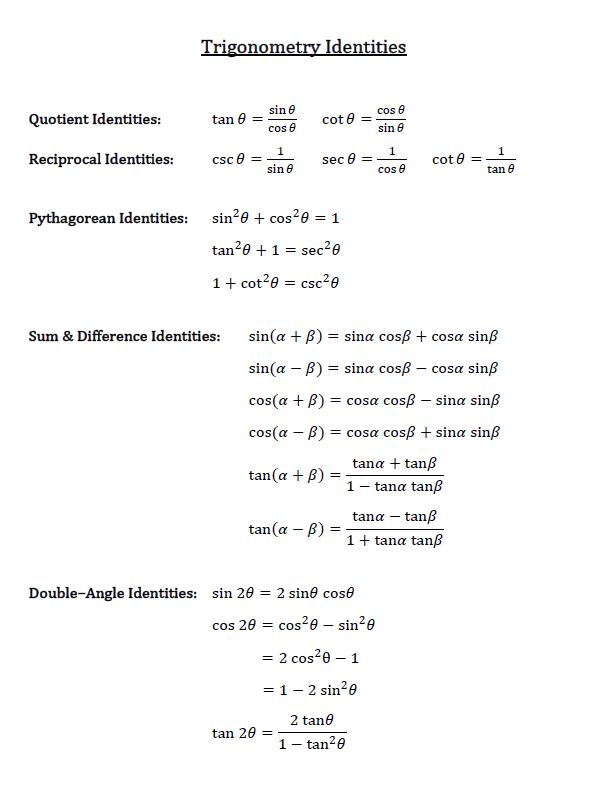# Sum and difference identities

### Sum and difference identities

Trig identities are formulas developed based on Pythagorean Theorem. These identities show us how and where to find the sine, cosine, and tangent of the sum and difference of two given angles.

#### Lessons

Download the Trigonometry identities chart hereFormulas:
$\sin (A + B)$
$\sin (A - B)$
$\cos (A + B)$
$\cos (A - B)$
$\tan (A + B)$
$\tan (A - B)$
• 1.
Simplify expressions

a)
sin 24°cos 36° + cos 24°sin 36°

b)
$\frac{tan {2 \pi \over 5 } - tan {3 \pi \over 20}}{1 + \tan {2 \pi \over 5} \cdot \tan {3 \pi \over 20}}$

• 2.
Prove Identities
a)
$\frac{\sin (A - B)}{\sin B} + \frac{\cos (A - B)}{\cos B} = \frac{\sin A}{\sin B \cos B}$

b)
$\frac{1 + \tan A}{\tan (A + {\pi \over 4})} = 1 - \tan A$

• 3.
Without using a calculator, evaluate:
a)
sin 15°

b)
sec (-105°)

c)
$tan {19\pi \over 12}$

• 4.
Given $\sin A = -{4 \over5}$ and $\cos B = {12 \over 13} ,$
where $\pi \leq A \leq {3 \pi \over 2}$ and ${3 \pi \over 2} \leq B \leq 2\pi ,$
find the exact value of $\cos (A + B)$